# High School Chemistry : Help with Concentration Units

## Example Questions

### Example Question #1 : Concentration And Units

What is the concentration of Ca in a solution of 1 mol CaCl2 in 1 L of distilled water? (M = molarity, m= molality)

Cannot be determined
2 M
1 m
2 m
1 M
Explanation:

The definition of molality is moles of solute in 1 kg of the solvent, whereas molarity is the number of moles of solute per 1 L of solutioin. Since 1 mol of CaCl2 is added to 1 L of water, this means that the volume of the final solution is greater than 1 L. Thus, molality is the more accurate concentration determinant, since the solution is probably close to 1 L.

### Example Question #1 : Concentration And Units

Which of the following is equivalent to molarity?

Moles of solute per liter of solution

Moles of solute per kilogram of solvent

Kilograms of solute per mole of solution

Moles of solute per liter of solvent

Moles of solute per liter of solution

Explanation:

Molarity, molality, and normality are the three principle ways to measure concentration. Molarity is a measure of moles of solute per liter of solution. Molality is a measure of moles of solute per kilogram of solvent. Normality expressly relates to acids and bases, and is the measure of moles of solute divided by the number of hydrogen equivalents per mole, all divided by liters of solution. Normality is also referred to as "equivalents (of acid) per liter."

### Example Question #1 : Help With Concentration Units

How many moles of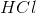are in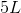of a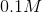solution?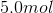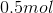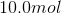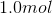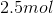Explanation:

Molarity is defined as moles of solute divided into liters of solution. We can set up the equation as follows: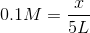Set the left side of the equation overand solve as a proportion.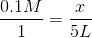Cross multiply.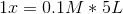Remember molarity is defined as moles of solute per liter of solution.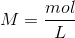Substiute.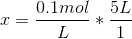Liters cancel out.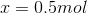Solve for the number of moles. This gives usof solute in asolution of.

### Example Question #1 : Help With Concentration Units

Which of the following units is common for measuring concentration?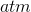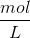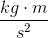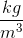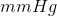Explanation:is the dimensional breakdown for the commonly used unit, or molarity, for concentration.

While the other units might be seen in chemistry, they are used for other topics.

Millimeters of mercury (mmHg) and atmospheres (atm) are seen in regards to pressure.is the dimensional breakdown for density. Andis the dimensional breakdown for a Newton, also written as N. This is associated with force.

### All High School Chemistry Resources